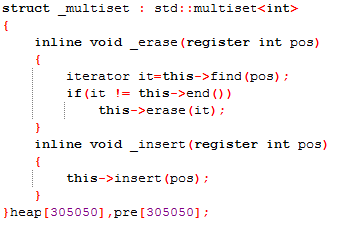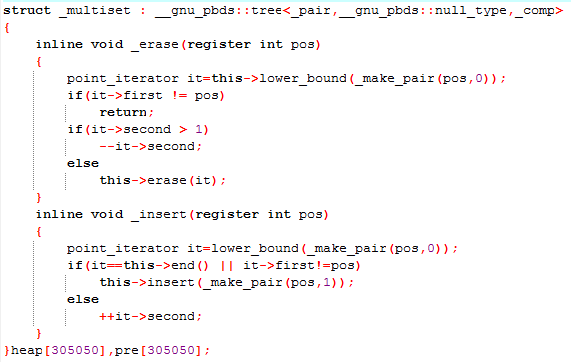# YZOJ P3706 [APIO2018]新家

• ### 样例 1 说明

• 对于第一个询问：小明在第 $$3$$ 年住在坐标为 $$5$$ 的地方。这一年中，编号为 $$1$$ 和 $$2$$ 的商店在营业， 到编号为 $$1$$ 的商店的距离为 $$2$$ ，到编号为 $$2$$ 的商店距离为 $$4$$ ，所以最大距离为 $$4$$。

• 对于第二个询问：小明在第 $$6$$ 年住在坐标为 $$5$$ 的地方。这一年中，编号为 $$1$$ 和 $$3$$ 的商店在营业， 到编号为 $$1$$ 的商店的距离为 $$2$$ ，到编号为 $$3$$ 的商店距离为 $$2$$ ，所以最大距离为 $$2$$。

• 对于第三个询问：小明在第 $$9$$ 年住在坐标为 $$5$$ 的地方。这一年中，编号为 $$1$$ 和 $$4$$ 的商店在营业， 它们的类型都为 $$1$$，没有类型为 $$2$$ 的商店在营业，所以答案为 $$-1$$。

• 同样的情况出现在第四个询问中。

$$[l, r]$$ 中存在所有类型的商店，其实等价于 $$\min\limits_{x=r+1}^{+\infty}{pre_x} \geq l$$ ，因为 $$(pre_x, x)$$ 中不存在这种类型的商店。

$$pre$$ 使用全局线段树维护（动态开点/离散化 均可），由于有删除（修改）操作，所以对每个叶子结点维护一个可删堆（或 multiset）。